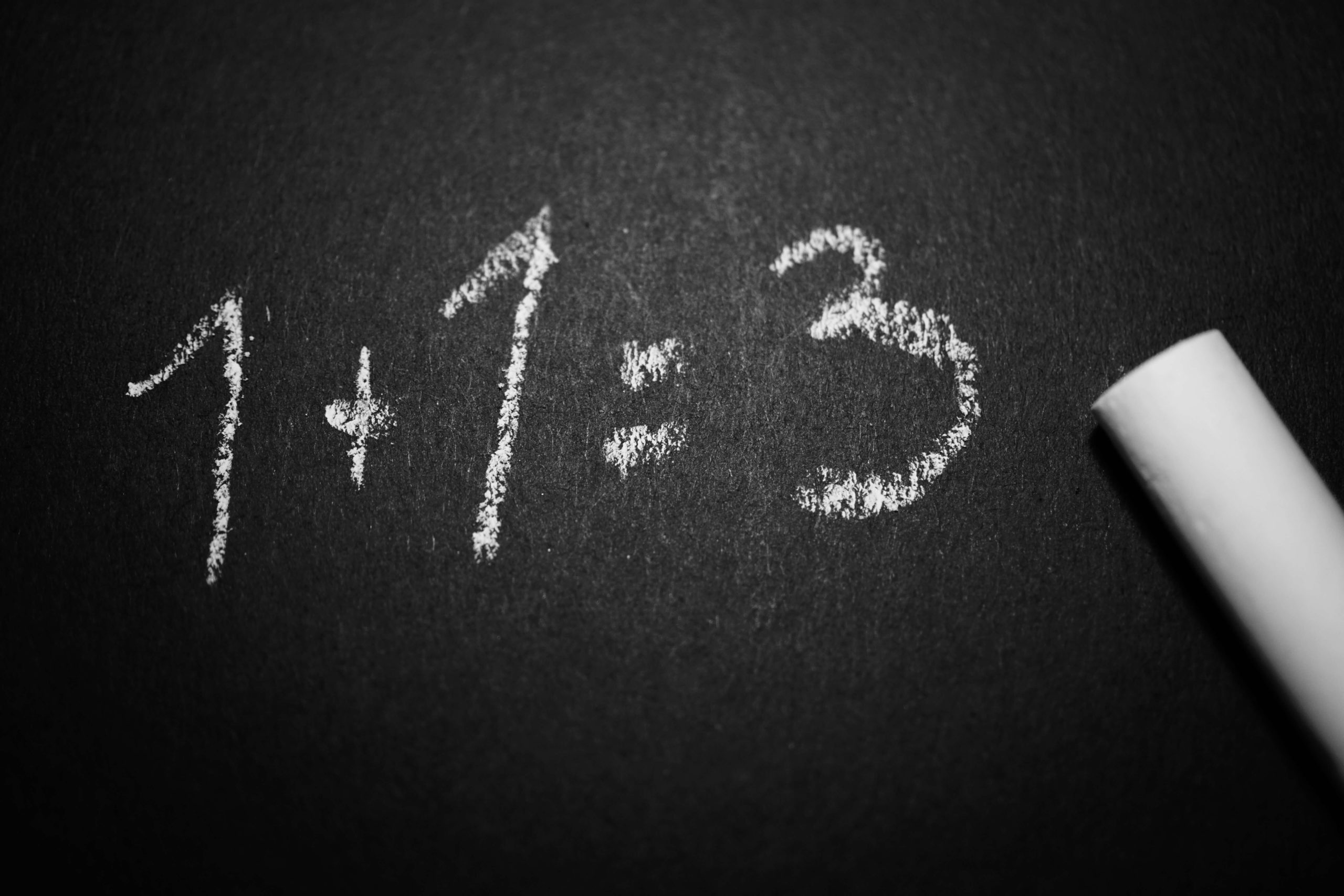# Fundamental Math Skills for Meat Processing

This course promises to provide you with an insightful use of mathematics in the management of the needs of everyday living to become a self-managing person.  It will equip you to use mathematics to plan and control personal, regional and/or national budgets and income and expenditure.

This course promises to provide you an insightful use of mathematics in the management of the needs of everyday living to become a self-managing person.  It will equip you to use mathematics to plan and control personal, regional and/or national budgets and income and expenditure.

• Convert numbers between the decimal number system and binary number system
• Work with numbers in different ways to express size/magnitude.
• Demonstrate the effect of error in calculations.
• Measure, estimate, and calculate physical quantities in practical situations relevant to the adult in life or the workplace
• Explore describe and represent, interpret and justify geometrical relationships and conjectures to solve problems in two and three dimensional geometrical situations.
• Pose questions, collect and organise data.
• Represent and interpret data using various techniques to investigate real life and work problems.
• Use random events to explore and apply probability concepts in simple life and work related situations.
• Use mathematics to plan and control personal and/or household budgets and income and expenditure.
• Use simple and compound interest to make sense of and define a variety of situations including investments, stokvels, inflation, appreciation and depreciation.
• Investigate various aspects of financial transactions including costs, prices, revenue, cost price, selling price, loss and profit.

### Resources

1
Meat Processing SP 1: Mathematics

### Unit Standard 9013

1
Lesson 1: Basic Geometric Shapes and Transformations
7:12
2
Lesson 2: Properties of Geometric Figures and Solids
6:44
3
Lesson 3: Scales and Maps
6:51
4
Lesson 4: Solve Problems with Formulae
5:36
5
Lesson 5: Solve Problems with Formulae: Part B
6:54
6
Lesson 6: Views of Objects
3:46
7
Quiz 1: Objects in Measurements
10 questions

### Unit Standard 9012

1
Lesson 1: Data
11:18
2
Lesson 2: Statistics
4:50
3
Lesson 3: Statistical Basics
3:30
4
Lesson 4: Data Collection
12:42
5
Lesson 5: Data Collection Methods
4:29
6
Quiz 1: Collecting, Organising and Representing Data
10 questions
7
Lesson 6: Probability Models
2:08
8
Lesson 7: Measurement
4:18
9
Lesson 8: Statistical Calculations
5:20
10
Lesson 9: Measures of Dispersion
10:43
11
Quiz 2: Probability Models
11 questions
12
Lesson 10: Probability
12:15
13
Lesson 11: Conditional Probability
3:20
14
Lesson 12: Independent vs Dependent Events
2:31
15
Lesson 13: Calculating Probability
15:15
16
Lesson 14: Probability and Statistical Models
6:08
17
Lesson 15: Full Picture of the Data
6:43
18
Quiz 3: Probability and Statistical Models
8 questions

### Unit Standard 7465

1
Lesson 1: Budget
7:39
2
Lesson 2: Budgeting Process
3:13
3
Lesson 3: Terminology
4:41
4
Quiz 1: Using Mathematics for Financial Instruments
8 questions
5
Lesson 4: Simple and Compound Interest
9:39
6
Lesson 5: Interest Rates
5:14
7
Lesson 6: Time Value of Money
3:50
8
Lesson 7: Annuity
6:19
9
Lesson 8: Futures
14:59
10
Lesson 9: Investing in Shares
8:53
11
Lesson 10: Pooled Investments
8:27
12
Lesson 11: RA’s vs Endowments
7:31
13
Quiz 2: Simple & Compound Interest
15 questions
14
Lesson 12: Profit Maximisation
2:46
15
Lesson 13: Marginal Revenue
9:19
16
Lesson 14: Marginal Cost
5:14
17
Lesson 15: Exchange Rates
7:37
18
Lesson 16: Currency in Relation to Remuneration
2:14
19
Lesson 17: Monetary Policy
6:13
20
Quiz 4: National & Global Economy
5 questions

#### Be the first to add a review.